/

### Blending Independent Components and Principal Components Analysis 2.5 Projection pursuit

Next page

2.5          Projection pursuit

Suppose we focus further on the property of non-normality, which we might measure via the (excess) kurtosis of a distribution. Kurtosis has two properties relevant to ICA:

(a)    All linear combinations of independent distributions have a smaller kurtosis than the largest kurtosis of any of the individual distributions (a result that can be derived using the Cauchy-Schwarz inequality).

(b)   Kurtosis is invariant to scalar multiplication, i.e. if the kurtosis of distributionis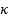then the kurtosis of the distribution defined by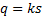where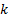is constant is also.

Suppose we also want to identify the input signals (up to a scalar multiple) one at a time, starting with the one with the highest kurtosis. This can be done via projection pursuit.

Given (a) and (b) we can expect the kurtosis of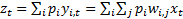to be maximised when this results in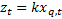for the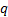corresponding to the signal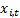which has the largest kurtosis, whereis arbitrary. Without loss of generality, we can reorder the input signals so that this one is deemed the first one, and thus we expect the kurtosis of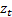to be maximised with respect to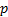when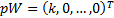and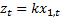. Although we do not at this stage know the full form of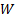we have still managed to extract out one signal (namely the one with the largest kurtosis) and found out something about.

In principle, the appropriate value ofcan be found using brute force exhaustive search, but in practice more efficient gradient based approaches would be used instead, see Section 2.8.

We can then remove the recovered source signal from the set of signal mixtures and repeat the above procedure to recover the next source signal from the ‘reduced’ set of signal mixtures. Repeating this iteratively, we should extract all available source signals (assuming that they are all lepto-kurtotic, i.e. all have kurtosis larger than any residual noise, which we might assume is merely normally distributed). The removal of each recovered source signal involves a projection of an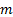-dimensional space onto one with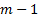dimensions and can be carried out using Gram-Schmidt orthogonalisation.

With any blind source separation method, a fundamental issue that has no simple answer is when to truncate such a search. If the mixing processes were noise free then the truncation should stop having extracted exactly the right number of signals (as long as there are at least as many distinct output signals as there are input signals). However, outputs are rarely noise free. In practice, therefore, we might truncate the signal search once the signals we seem to be extracting via it appear to be largely artefacts of noise in the signals or mixing process, rather than suggestive of additional true underlying source signals.

We see similarities with random matrix theory, an approach used to truncate the output of a principal components analysis to merely those principal components that are probably not just artefacts of noise in the input data.